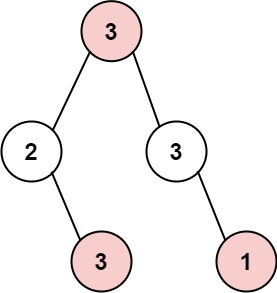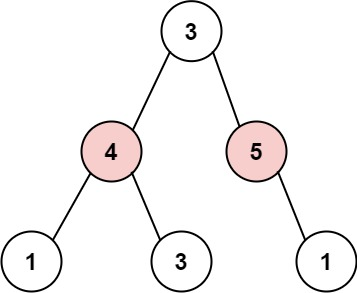# GeetCode Hub

The thief has found himself a new place for his thievery again. There is only one entrance to this area, called `root`.

Besides the `root`, each house has one and only one parent house. After a tour, the smart thief realized that all houses in this place form a binary tree. It will automatically contact the police if two directly-linked houses were broken into on the same night.

Given the `root` of the binary tree, return the maximum amount of money the thief can rob without alerting the police.

Example 1:```Input: root = [3,2,3,null,3,null,1]
Output: 7
Explanation: Maximum amount of money the thief can rob = 3 + 3 + 1 = 7.
```

Example 2:```Input: root = [3,4,5,1,3,null,1]
Output: 9
Explanation: Maximum amount of money the thief can rob = 4 + 5 = 9.
```

Constraints:

• The number of nodes in the tree is in the range `[1, 104]`.
• `0 <= Node.val <= 104`

/** * Definition for a binary tree node. * public class TreeNode { * int val; * TreeNode left; * TreeNode right; * TreeNode() {} * TreeNode(int val) { this.val = val; } * TreeNode(int val, TreeNode left, TreeNode right) { * this.val = val; * this.left = left; * this.right = right; * } * } */ class Solution { public int rob(TreeNode root) { } }